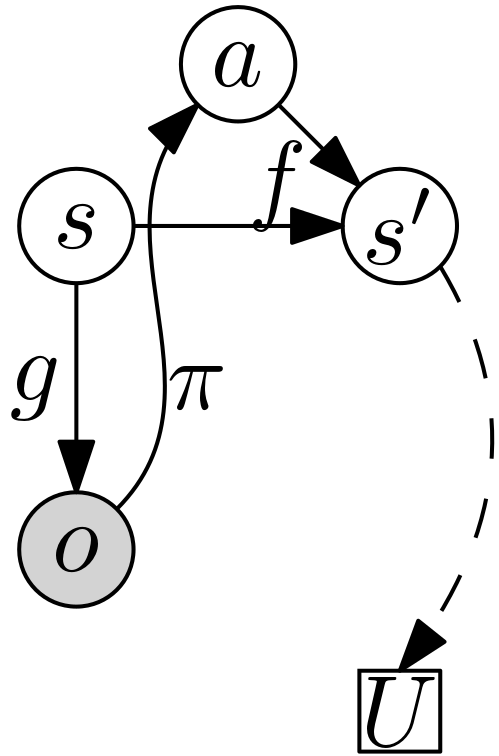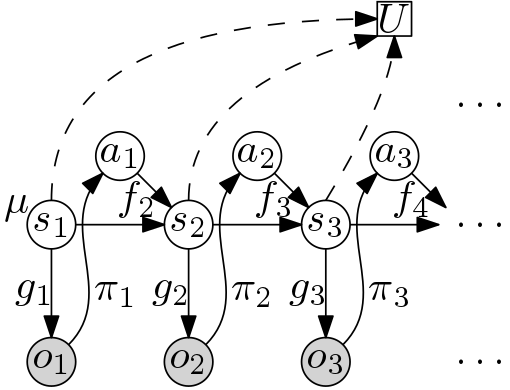# probabilistic view of ai

10 March 2017

this note is about formulating the problem of solving ai (ai problem) from a perspective of combining probabilistic inference and decision theory. it’s trying to present a coherent picture of the ai problem and how various subfields of machine learning fit into it and how they approximate solutions to this problem. the hope is to identify and prioritize interesting topics for research. this is heavily based on chapters 16, 17 and 21 of the ai book (Russell & Norvig, 2010).

## 1 non-sequential decision problemgraphical model of the non-sequential decision problem. square means a decision node.

### 1.1 definitions

• probability triple
• $$(\Omega, \mathcal F, \mathbb P)$$,
• current state of the world is a random variable
• $$S: \Omega \to \mathcal S$$, taking values $$s \in \mathcal S$$,
• observation of the world made by the agent is a random variable
• $$O: \Omega \to \mathcal O$$, taking values $$o \in \mathcal O$$,
• next state of the world is a random variable
• $$S': \Omega \to \mathcal S'$$, taking values $$s' \in \mathcal S'$$,
• action taken by the agent in the current time is a random variable
• $$A: \Omega \to \mathcal A$$, taking values $$a \in \mathcal A$$,
• utility function describes the desirability of the state
• $$U: \mathcal S' \to \mathbb R$$.

### 1.2 expected utility of an action

expected utility of an action is a function $$EU: \mathcal A \times \mathcal O \to \mathbb R$$: \begin{align} EU(a, o) = \E[\underbrace{U(S’ \given A = a, O = o)}_{\text{random variable, } \Omega \to \mathbb R}]. \end{align}

### 1.3 maximum expected utility principle

the maximum expected utility principle says that, given an observation $$o$$, a rational agent should choose the action $$a^\ast$$ that maximizes the agent’s expected utility: \begin{align} a^\ast = \argmax_{a} EU(a, o). \end{align}

why this makes sense is philosophical. checkout discussion in notes on utility theory.

the quantities needed to evaluate $$EU(a, o)$$ are

• world model:
• prior,
• transition,
• emission,
• agent’s model:
• policy,
• next state posterior.

### 1.4 world model (prior)

• probability distribution $$\mathcal L(S): \mathcal S \to \mathbb R$$,
• probability density $$\mu(s)$$.

### 1.5 world model (transition)

• probability distribution $$\mathcal L(S' \given A = a, S = s)$$,
• probability density $$f(s' \given a, s)$$.

### 1.6 world model (emission)

• probability distribution $$\mathcal L(O \given S = s)$$
• probability density $$g(o \given s)$$.

### 1.7 agent’s model (policy)

• probability distribution $$\mathcal L(A \given O = o)$$,
• probability density $$\pi(a \given o)$$.

### 1.8 next state posterior

• probability distribution $$\mathcal L(S' \given A = a, O = o)$$,
• probability density: \begin{align} h(s’ \given a, o) = \int_{\mathcal S} f(s’ \given a, s) \pi(a \given o) g(o \given s) \mu(s) \,\mathrm ds. \end{align}

### 1.9 expected utility of an action

finally, \begin{align} EU(a, o) &= \E[U(S’ \given A = a, O = o)] \\
&= \int_{\mathcal S’} U(s’) h(s’ \given a, o) \,\mathrm ds’ \\
&= \int_{\mathcal S’} U(s’) \left[ \int_{\mathcal S} f(s’ \given a, s) \pi(a \given o) g(o \given s) \mu(s) \,\mathrm ds \right]\,\mathrm ds’ \end{align}

now we turn into the full sequential problem…

## 2 sequential decision problemgraphical model of the sequential decision problem. square means a decision node.

### 2.1 definitions

• probability triple
• $$(\Omega, \mathcal F, \mathbb P)$$,
• state of the world at time $$t$$ is a random variable
• $$S_t: \Omega \to \mathcal S_t$$, taking values $$s_t \in \mathcal S_t$$,
• observation of the world made by the agent at time $$t$$ is a random variable
• $$O_t: \Omega \to \mathcal O_t$$, taking values $$o_t \in \mathcal O_t$$,
• action taken by the agent after observing it at time $$t$$ is a random variable
• $$A_t: \Omega \to \mathcal A_t$$, taking values $$a_t \in \mathcal A_t$$,
• utility function describes the desirability of the state
• $$U: \times_{t = 1}^{\infty} \mathcal S_t \to \mathbb R$$.

we’ll define

• world model:
• prior,
• transition,
• emission,
• agent’s model:
• policy,
• next state posterior,
• all states posterior,
• future states posterior.

### 2.2 world model (prior)

• probability distribution $$\mathcal L(S_1)$$,
• probability density $$\mu(s_1)$$.

### 2.3 world model (transition)

• probability distribution $$\mathcal L(S_t \given A_{t - 1} = a_{t - 1}, S_{t - 1} = s_{t - 1})$$,
• probability density $$f_t(s_t \given a_{t - 1}, s_{t - 1})$$.

### 2.4 world model (emission)

• probability distribution $$\mathcal L(O_t \given S_t = s_t)$$,
• probability density $$g_t(o_t \given s_t)$$.

### 2.5 agent’s model (policy)

• probability distribution $$\mathcal L(A_t \given O_t = o_t)$$
• probability density $$\pi_t(a_t \given o_t)$$

### 2.6 next state posterior

• probability distribution $$\mathcal L(S_{t + 1} \given A_t = a_t, O_t = o_t, \dotsc, A_1 = a_1, O_1 = o_1)$$,
• probability density:
• define $$h_1(s_1) = \mu(s_1)$$,
• for $$t = 1, 2, \dotsc$$: \begin{align} &h_{t + 1}(s_{t + 1} \given a_t, o_t, \dotsc, a_1, o_1) \\
&= \frac{\int_{\mathcal S_t} f_{t + 1}(s_{t + 1} \given a_t, s_t) \pi_t(a_t \given o_t) g_t(o_t \given s_t) h_t(s_t \given a_{t - 1}, o_{t - 1}, \dotsc, a_1, o_1) \,\mathrm ds_t}{\int_{\mathcal S_t \times \mathcal S_{t + 1}} f_{t + 1}(s_{t + 1} \given a_t, s_t) \pi_t(a_t \given o_t) g_t(o_t \given s_t) h_t(s_t \given a_{t - 1}, o_{t - 1}, \dotsc, a_1, o_1) \,\mathrm ds_t \,\mathrm ds_{t + 1}}. \end{align}

### 2.7 all states posterior

• probability distribution $$\mathcal L(S_1, S_2, \dotsc \given A_1 = a_1, O_1 = o_1)$$,
• probability density: \begin{align} &k(s_1, s_2, \dotsc \given a_1, o_1) \\
&= \frac{\int_{\mathcal A_{2:\infty} \times \mathcal O_{2:\infty}} \mu(s_1) \prod_{t = 1}^\infty g_t(o_t \given s_t) \pi_t(a_t \given o_t) f_{t + 1}(s_{t + 1} \given a_t, s_t) \,\mathrm da_{2:\infty} \,\mathrm do_{2:\infty}}{\int_{\mathcal S_{1:\infty} \times \mathcal A_{2:\infty} \times \mathcal O_{2:\infty}} \mu(s_1) \prod_{t = 1}^\infty g_t(o_t \given s_t) \pi_t(a_t \given o_t) f_{t + 1}(s_{t + 1} \given a_t, s_t) \,\mathrm ds_{1:\infty} \,\mathrm da_{2:\infty} \,\mathrm do_{2:\infty}} \end{align}

## 4 bayesian (model-based) reinforcement learning

1. Russell, S. J., & Norvig, P. (2010). Artificial Intelligence (A Modern Approach). Prentice Hall.
@misc{russell2010artificial,
title = {Artificial Intelligence (A Modern Approach)},
author = {Russell, Stuart J and Norvig, Peter},
year = {2010},
publisher = {Prentice Hall}
}


[back]